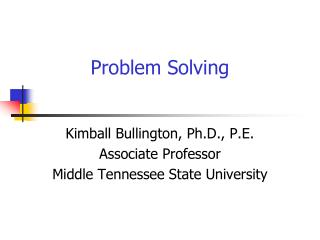# Problem Solving - PowerPoint PPT PresentationDownload PresentationProblem Solving

Problem SolvingDownload Presentation## Problem Solving

- - - - - - - - - - - - - - - - - - - - - - - - - - - E N D - - - - - - - - - - - - - - - - - - - - - - - - - - -
##### Presentation Transcript

1. Problem Solving Kimball Bullington, Ph.D., P.E. Associate Professor Middle Tennessee State University

2. Where are we going? • What is a problem anyway? • Problem Solving Methodologies • Using DMAIC as a Model • One Powerful Technique

3. Problem What is a problem, anyway?

4. What is a problem anyway? • prob·lem/ˈpräbləm/Noun: 1.A matter or situation regarded as unwelcome or harmful and needing to be dealt with and overcome. • 2.A thing difficult to achieve or accomplish.

5. What is a problem anyway? • Can we separate problems from causes and solutions? It isn’t easy.

6. Some Problems are Difficult • Lack of clarity • Multiple goals • Complexity • Dynamics

7. Some Problems are Difficult • Lack of clarity • Multiple goals • Complexity • Dynamics

8. Where are we going? • What is a problem anyway? • Problem Solving Methodologies • Using DMAIC as a Model • One Powerful Technique

9. Problem Solving Methodologies • Kepner / Tregoe • GROW • How to Solve It • Productive Thinking • TIPS (TRIZ) • PDCA / DMAIC Wikipedia, 12/13/11

10. Kepner / Tregoe • The Rational Manager • Problem analysis • Decision making • Potential problem analysis • Direction and Control

11. GROW • Goals • Reality • Obstacles / Options • Way forward Example: weight loss Wikipedia, 12/13/11

12. Problem Solving Methodologies • Kepner / Tregoe • GROW • How to Solve It • Productive Thinking • TIPS (TRIZ) • PDCA / DMAIC

13. How to Solve it: 4 Principles • Understand the problem • Devise a plan • Carry out the plan • Review / extend How to Solve It: George Polya Wikipedia, 12/13/11

14. Productive Thinking: 6 Steps • What’s going on? • What’s success? • What’s the question? • Generate answers • Forge the solution • Align resources Wikipedia, 12/13/11

15. TIPS / TRIZ • Theory of Inventive Problem Solving • Specific Problem • Typical Problem (contradiction) • Typical Solution • Specific Solution • Inventive principles and standards

16. Where are we going? • What is a problem anyway? • Problem Solving Methodologies • Using DMAIC as a Model • One Powerful Technique

17. PDCA / DMAIC

18. DMAIC • Define • Measure • Analyze • Improve • Control

19. Lean or Six Sigma? • Lean • Flow • Waste • Six Sigma • Variability • Defect • Implementation

20. Why Four Sigma? • Most businesses are in the three to four sigma range • Can’t jump to six sigma • 80 / 20 rule • Simple first, complex later

21. Where are we going? • What is a problem anyway? • Problem Solving Methodologies • Using DMAIC as a Model • One Powerful Technique

22. SWOT Analysis

23. SWOT Analysis

24. SWOT Analysis

25. SWOT Analysis

26. Take-aways • What is a problem anyway? • Problem Solving Methodologies • Using DMAIC as a Model • Using CED’s to better define your problem causes

27. References Books: • Six Sigma Pocket Guide (Rath & Strong’s) • The Black Belt Memory Jogger (GOAL / QPC) • The New Rational Manager (Kepner & Tregoe) • How to Solve It (George Polya) • And Suddenly the Inventor Appeared (Genrich Altshuller) • Trizics (Gordon Cameron) Web sites: • www.isixsigma.com • http://en.wikipedia.org/wiki/Problem_solving